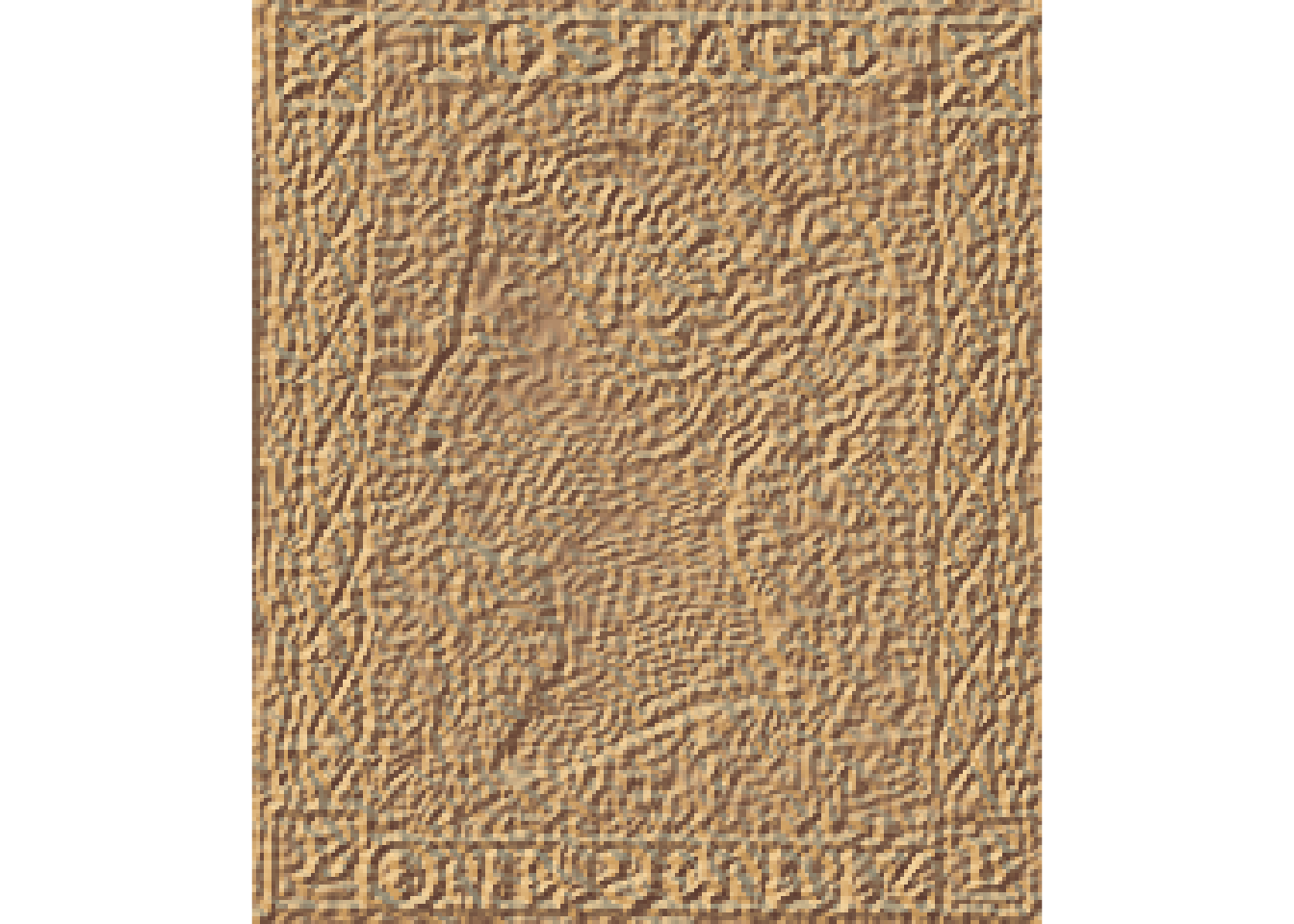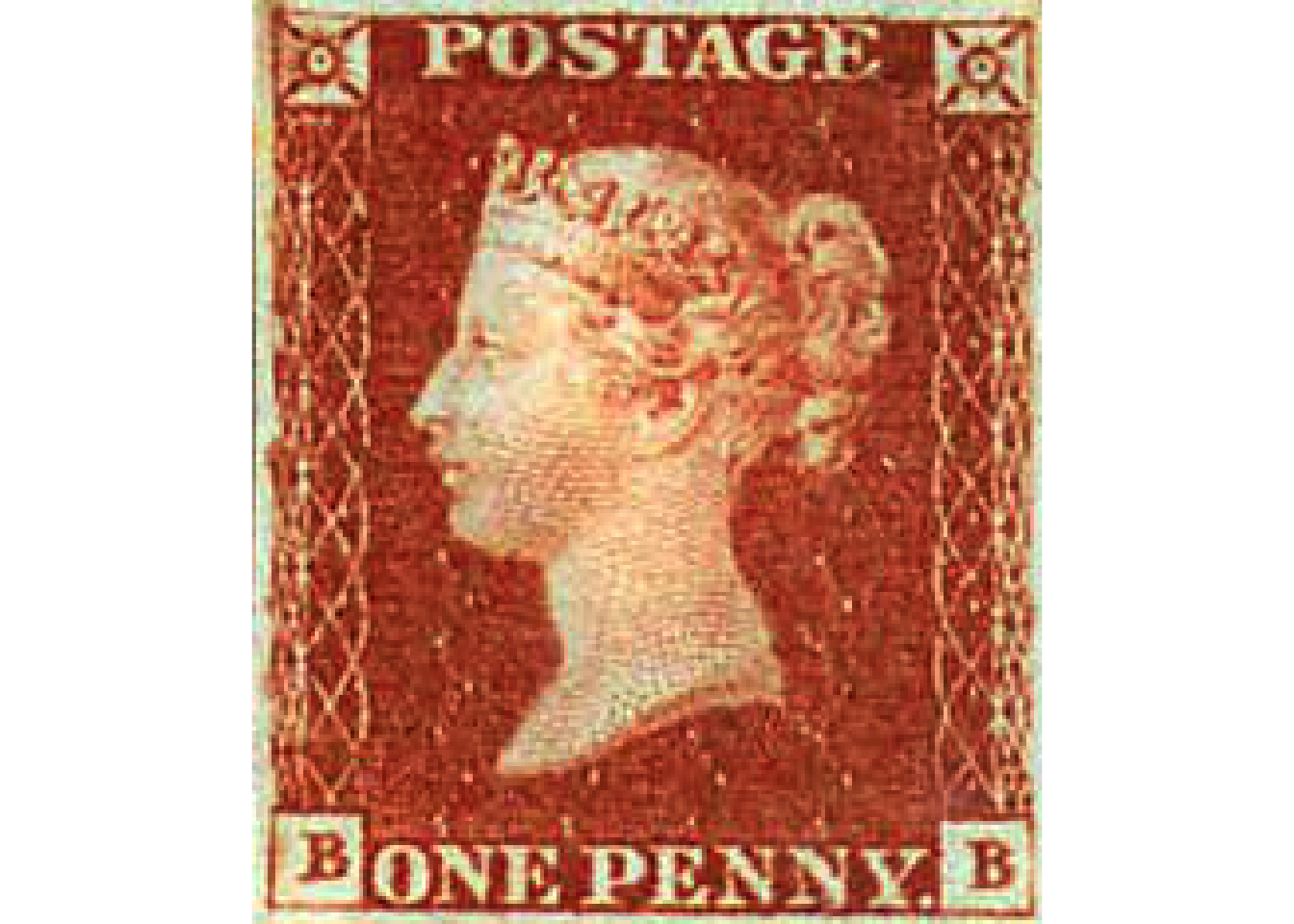# An Aside - The Penny Red

Although our main focus in using `rayshader`’s 3D plotting capabilities will be to render 3D interactive maps, it’s worth bearing in mind that `rayshader` can be used as a general purpose 3D visualisation tool.

So before we start rendering 3D maps, and before we are tempted to think that maps are the only thing we can plot with `rayshader`, let’s visualise something completely different: the colour of a Penny Red stamp…

We can obtain an appropriate image file from Wikipedia and save a local copy of it:

``````image.url = 'https://upload.wikimedia.org/wikipedia/commons/a/a4/PennyRed.jpeg'
pennyred_file = 'PennyRed.jpeg'

# Download the file from a specified web location to a specifically named file

## 3D Rendering of Colour Images Using `rayshader`

To use `rayshader` to render the image file, we need to obtain some “elevation” levels that will project some attribute of the image into the vertical z dimension.

One obvious candidate is the RGB colour value (we might alternatively render just the red, green or blue components) mapped to a single elevation value by regarding it as a base 256 encoded value:

``````library(raster)

# Create a raster file from the image
pennyred = raster(pennyred_file)

# Isolate the reg, green and blue components
pennyred_red = pennyred_image[,,1]
pennyred_green = pennyred_image[,,2]
pennyred_blue = pennyred_image[,,3]

#https://www.maptiler.com/news/2019/03/rgb-encoded-elevation-data-in-maptiler-cloud/
# height = -10000 + ((R * 256 * 256 + G * 256 + B) * 0.1)

# Use the RGB values as a base 256 elevation encoding
# then reduce the height with a base value
# We should really calculate the base value rather than use a
# value determined by observation...
# The values() function sets the value of the pennyred raster
values(pennyred) = -16700 + (((255-pennyred_red ) * 256 * 256 +
(255-pennyred_green ) * 256 +
(255-pennyred_blue)) * 0.001)

# The pennyred raster now has values that encode RGB-as-elevation
pennyred``````
``````## class      : RasterLayer
## band       : 1  (of  3  bands)
## dimensions : 234, 200, 46800  (nrow, ncol, ncell)
## resolution : 1, 1  (x, y)
## extent     : 0, 200, 0, 234  (xmin, xmax, ymin, ymax)
## crs        : NA
## source     : memory
## names      : PennyRed
## values     : 11.42209, 58.69265  (min, max)``````

To create an elevation matrix from the data, we might consider scaling from the raw RGB values:

``````elev_matrix_pennyred <- matrix(
raster::extract(pennyred, raster::extent(pennyred)),
nrow = ncol(pennyred), ncol = nrow(pennyred)
)``````

The `rayshader` package takes a simple elevation matrix and renders it in 2 or 3 dimensional relief.

For example, here’s a simple 2D rendering:

``````library(rayshader)

elev_matrix_pennyred %>%
plot_map()````````  #plot_3d(elev_matrix_pennyred)``

We can also add the original image back as an overlay. In this case, we lose the 3D effect:

``````rayshaded_penny_red = elev_matrix_pennyred %>%

plot_map()``````However, if we view the elevated image in a 3D plot, we can see the elevation map far more clearly:

``````# Configuration settings to allow us to render the WebGL
options(rgl.useNULL = TRUE,
rgl.printRglwidget = TRUE)

rgl::clear3d()